Last updated: 2018-11-23

• R Markdown file: up-to-date

Great! Since the R Markdown file has been committed to the Git repository, you know the exact version of the code that produced these results.

• Environment: empty

Great job! The global environment was empty. Objects defined in the global environment can affect the analysis in your R Markdown file in unknown ways. For reproduciblity it’s best to always run the code in an empty environment.

• Seed: set.seed(20180730)

The command set.seed(20180730) was run prior to running the code in the R Markdown file. Setting a seed ensures that any results that rely on randomness, e.g. subsampling or permutations, are reproducible.

• Session information: recorded

Great job! Recording the operating system, R version, and package versions is critical for reproducibility.

• Repository version: 7d8a1dd

Great! You are using Git for version control. Tracking code development and connecting the code version to the results is critical for reproducibility. The version displayed above was the version of the Git repository at the time these results were generated.

Note that you need to be careful to ensure that all relevant files for the analysis have been committed to Git prior to generating the results (you can use wflow_publish or wflow_git_commit). workflowr only checks the R Markdown file, but you know if there are other scripts or data files that it depends on. Below is the status of the Git repository when the results were generated:

Ignored files:
Ignored:    .DS_Store
Ignored:    .Rhistory
Ignored:    .Rproj.user/
Ignored:    analysis/cache.bak.20181031/
Ignored:    analysis/cache.bak/
Ignored:    analysis/cache.lind2.20181114/
Ignored:    analysis/cache/
Ignored:    data/Lindstrom2/
Ignored:    data/processed.bak.20181031/
Ignored:    data/processed.bak/
Ignored:    data/processed.lind2.20181114/
Ignored:    packrat/lib-R/
Ignored:    packrat/lib-ext/
Ignored:    packrat/lib/
Ignored:    packrat/src/

Unstaged changes:
Modified:   .gitignore
Modified:   output/04B_Organoids_Nephron/cluster_de.xlsx
Modified:   output/04B_Organoids_Nephron/conserved_markers.xlsx
Modified:   output/04B_Organoids_Nephron/markers.xlsx
Modified:   output/04_Organoids_Clustering/cluster_de.xlsx
Modified:   output/04_Organoids_Clustering/conserved_markers.xlsx
Modified:   output/04_Organoids_Clustering/markers.xlsx
Modified:   output/07B_Combined_Nephron/cluster_de.xlsx
Modified:   output/07B_Combined_Nephron/cluster_de_filtered.xlsx
Modified:   output/07B_Combined_Nephron/conserved_markers.xlsx
Modified:   output/07B_Combined_Nephron/markers.xlsx
Modified:   output/07_Combined_Clustering/cluster_de.xlsx
Modified:   output/07_Combined_Clustering/cluster_de_filtered.xlsx
Modified:   output/07_Combined_Clustering/conserved_markers.xlsx
Modified:   output/07_Combined_Clustering/markers.xlsx


Note that any generated files, e.g. HTML, png, CSS, etc., are not included in this status report because it is ok for generated content to have uncommitted changes.
Expand here to see past versions:
File Version Author Date Message
Rmd 7d8a1dd Luke Zappia 2018-11-23 Minor fixes to output
Rmd 91ebd58 Luke Zappia 2018-11-21 Move summariseClusts function to file
html 91ebd58 Luke Zappia 2018-11-21 Move summariseClusts function to file
html a61f9c9 Luke Zappia 2018-09-13 Rebuild site
html ad10b21 Luke Zappia 2018-09-13 Switch to GitHub
Rmd bff4d5b Luke Zappia 2018-08-14 Add crossover document

# Presentation
library("knitr")
library("glue")

# Paths
library("here")

# Tidyverse
library("tidyverse")
source(here("R/output.R"))
source(here("R/crossover.R"))
orgs.path <- here("output/04_Organoids_Clustering/cluster_assignments.csv")
orgs.neph.path <- here("output/04B_Organoids_Nephron/cluster_assignments.csv")
comb.path <- here("output/07_Combined_Clustering/cluster_assignments.csv")
comb.neph.path <- here("output/07B_Combined_Nephron/cluster_assignments.csv")

# Introduction

In this document we are going to load the results of the various clustering analyses and compare them. The goal is to see if they are consistent by checking that clusters in different analyses that have been assigned the same cell types actually contain the same cells.

orgs.clusts <- read_csv(orgs.path,
col_types = cols(
Cell = col_character(),
Dataset = col_character(),
Sample = col_integer(),
Barcode = col_character(),
Cluster = col_integer()
)) %>%
rename(Organoids = Cluster)

col_types = cols(
Cell = col_character(),
Dataset = col_character(),
Sample = col_integer(),
Barcode = col_character(),
Cluster = col_integer()
)) %>%
rename(OrgsNephron = Cluster)

col_types = cols(
Cell = col_character(),
Dataset = col_character(),
Sample = col_integer(),
Barcode = col_character(),
Cluster = col_integer()
)) %>%
rename(Combined = Cluster)

col_types = cols(
Cell = col_character(),
Dataset = col_character(),
Sample = col_integer(),
Barcode = col_character(),
Cluster = col_integer()
)) %>%
rename(CombNephron = Cluster)

clusts <- comb.clusts %>%
left_join(comb.neph.clusts,
by = c("Cell", "Dataset", "Sample", "Barcode")) %>%
left_join(orgs.clusts,
by = c("Cell", "Dataset", "Sample", "Barcode")) %>%
left_join(orgs.neph.clusts,
by = c("Cell", "Dataset", "Sample", "Barcode"))

We are going to do this using a kind of heatmap. Clustering results from two separate analyses will form the x and y axes and each cell will represent the overlap in samples between two clusters. We will colour the cells using the Jaccard index, a measure of similarity between to groups that is equal to the size of the intersect divided by the size of the union. This will highlight clusters that are particularly similar. We will also label cells with the proportion of samples in a cluster that are also in another, so that rows and columns will each sum to one (using a separate colour for each).

# Organoids

## vs Organoids Nephron

summariseClusts(clusts, Organoids, OrgsNephron) %>%
ggplot(aes(x = Organoids, y = OrgsNephron, fill = Jaccard)) +
geom_tile() +
geom_text(aes(label = round(OrganoidsPct, 2)), nudge_y = 0.2,
colour = "#ff698f", size = 6) +
geom_text(aes(label = round(OrgsNephronPct, 2)), nudge_y = -0.2,
colour = "#73b4ff", size = 6) +
geom_text(aes(label = glue("({OrganoidsTotal})")), y = -0.05,
size = 5, colour = "#ff698f") +
geom_text(aes(label = glue("({OrgsNephronTotal})")), x = -0.05,
size = 5, colour = "#73b4ff") +
scale_fill_viridis_c(begin = 0.02, end = 0.98, na.value = "black",
limits = c(0, 1)) +
coord_equal() +
expand_limits(x = -0.5, y = -0.5) +
labs(x = "Organoids cluster",
y = "Organoids nephron cluster",
caption = "Numbers in brackets show cluster size") +
theme_minimal() +
theme(axis.text = element_text(size = 20),
axis.text.x = element_text(colour = "#ff698f"),
axis.text.y = element_text(colour = "#73b4ff"),
axis.ticks = element_blank(),
axis.title = element_text(size = 15),
axis.title.x = element_text(colour = "#ff698f"),
axis.title.y = element_text(colour = "#73b4ff"),
legend.key.height = unit(50, "pt"),
legend.title = element_text(size = 15),
legend.text = element_text(size = 10),
panel.grid = element_blank())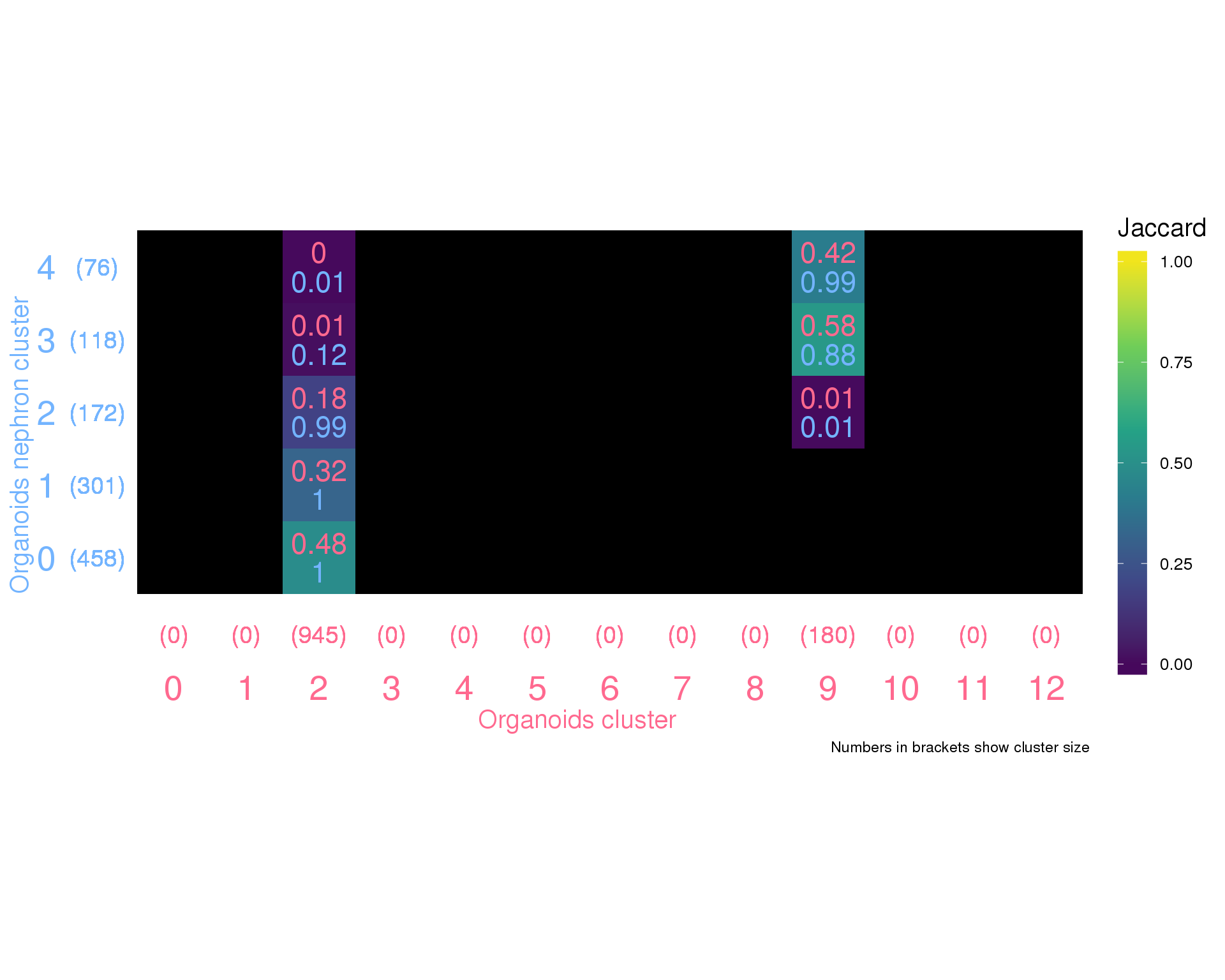Expand here to see past versions of orgs-orgs-neph-1.png:
Version Author Date
91ebd58 Luke Zappia 2018-11-21

## vs Combined

summariseClusts(clusts, Organoids, Combined) %>%
ggplot(aes(x = Organoids, y = Combined, fill = Jaccard)) +
geom_tile() +
geom_text(aes(label = round(OrganoidsPct, 2)), nudge_y = 0.2,
colour = "#ff698f", size = 4) +
geom_text(aes(label = round(CombinedPct, 2)), nudge_y = -0.2,
colour = "#73b4ff", size = 4) +
geom_text(aes(label = glue("({OrganoidsTotal})")), y = -0.05,
size = 3.5, colour = "#ff698f") +
geom_text(aes(label = glue("({CombinedTotal})")), x = -0.05,
size = 3.5, colour = "#73b4ff") +
scale_fill_viridis_c(begin = 0.02, end = 0.98, na.value = "black",
limits = c(0, 1)) +
coord_equal() +
expand_limits(x = -0.5, y = -0.5) +
labs(x = "Organoids cluster",
y = "Combined cluster",
caption = "Numbers in brackets show cluster size") +
theme_minimal() +
theme(axis.text = element_text(size = 20),
axis.text.x = element_text(colour = "#ff698f"),
axis.text.y = element_text(colour = "#73b4ff"),
axis.ticks = element_blank(),
axis.title = element_text(size = 15),
axis.title.x = element_text(colour = "#ff698f"),
axis.title.y = element_text(colour = "#73b4ff"),
legend.key.height = unit(50, "pt"),
legend.title = element_text(size = 15),
legend.text = element_text(size = 10),
panel.grid = element_blank())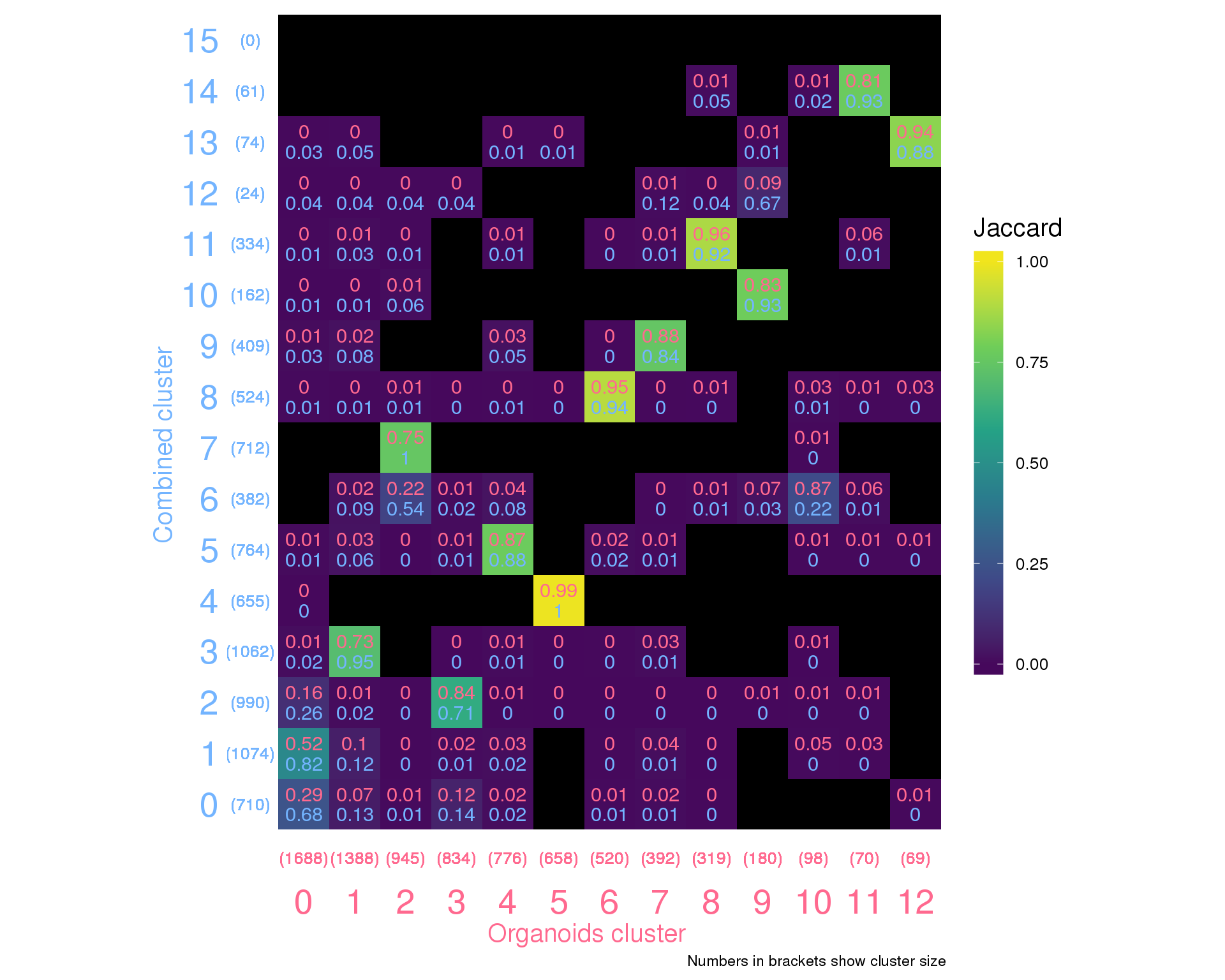Expand here to see past versions of orgs-combined-1.png:
Version Author Date
91ebd58 Luke Zappia 2018-11-21

## vs Combined Nephron

summariseClusts(clusts, Organoids, CombNephron) %>%
ggplot(aes(x = Organoids, y = CombNephron, fill = Jaccard)) +
geom_tile() +
geom_text(aes(label = round(OrganoidsPct, 2)), nudge_y = 0.2,
colour = "#ff698f", size = 6) +
geom_text(aes(label = round(CombNephronPct, 2)), nudge_y = -0.2,
colour = "#73b4ff", size = 6) +
geom_text(aes(label = glue("({OrganoidsTotal})")), y = -0.05,
size = 5, colour = "#ff698f") +
geom_text(aes(label = glue("({CombNephronTotal})")), x = -0.05,
size = 5, colour = "#73b4ff") +
scale_fill_viridis_c(begin = 0.02, end = 0.98, na.value = "black",
limits = c(0, 1)) +
coord_equal() +
expand_limits(x = -0.5, y = -0.5) +
labs(x = "Organoids cluster",
y = "Combined nephron cluster",
caption = "Numbers in brackets show cluster size") +
theme_minimal() +
theme(axis.text = element_text(size = 20),
axis.text.x = element_text(colour = "#ff698f"),
axis.text.y = element_text(colour = "#73b4ff"),
axis.ticks = element_blank(),
axis.title = element_text(size = 15),
axis.title.x = element_text(colour = "#ff698f"),
axis.title.y = element_text(colour = "#73b4ff"),
legend.key.height = unit(50, "pt"),
legend.title = element_text(size = 15),
legend.text = element_text(size = 10),
panel.grid = element_blank())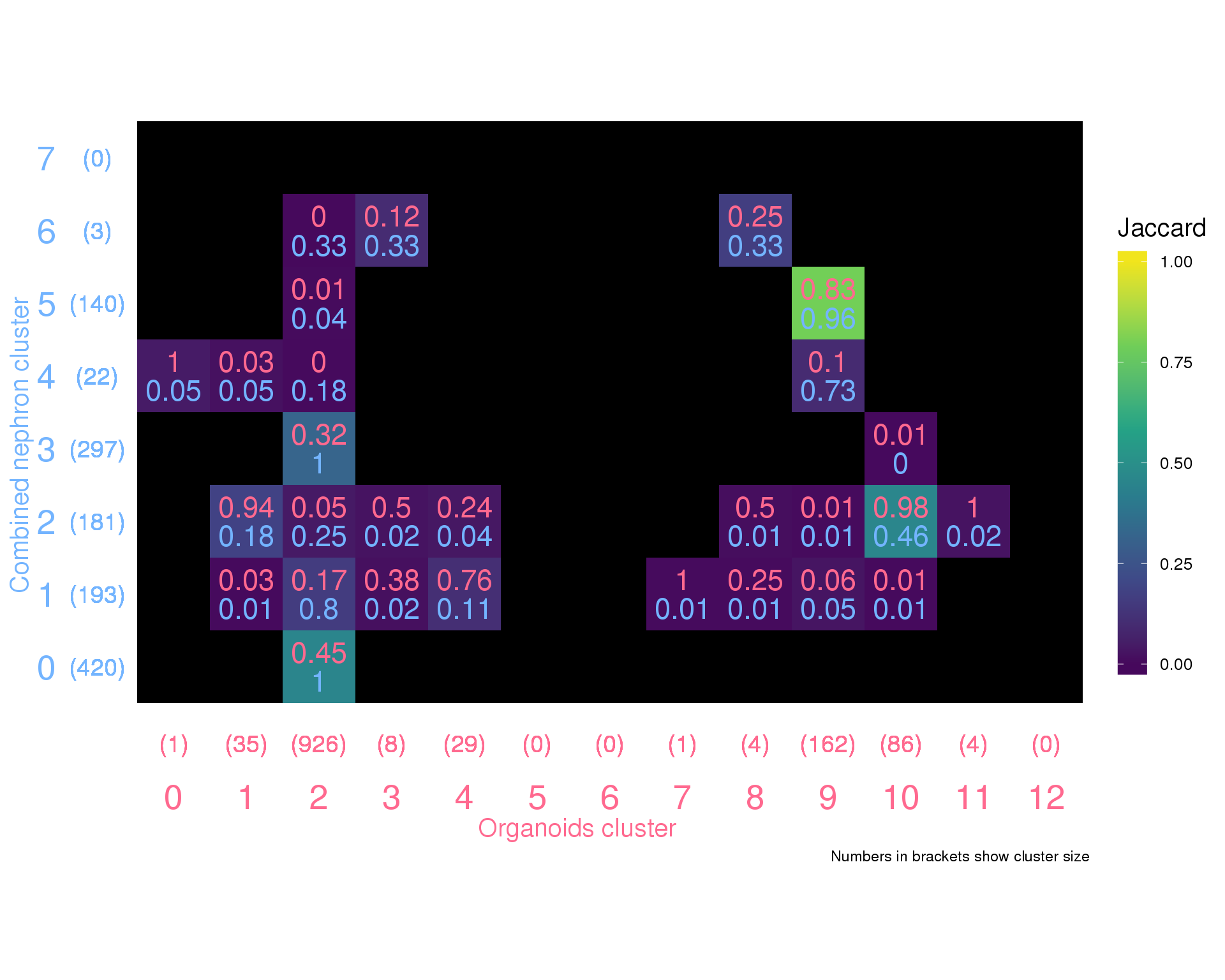Expand here to see past versions of orgs-comb-neph-1.png:
Version Author Date
91ebd58 Luke Zappia 2018-11-21

# Combined

## vs Combined Nephron

summariseClusts(clusts, Combined, CombNephron) %>%
ggplot(aes(x = Combined, y = CombNephron, fill = Jaccard)) +
geom_tile() +
geom_text(aes(label = round(CombinedPct, 2)), nudge_y = 0.2,
colour = "#ff698f", size = 6) +
geom_text(aes(label = round(CombNephronPct, 2)), nudge_y = -0.2,
colour = "#73b4ff", size = 6) +
geom_text(aes(label = glue("({CombinedTotal})")), y = -0.05,
size = 5, colour = "#ff698f") +
geom_text(aes(label = glue("({CombNephronTotal})")), x = -0.05,
size = 5, colour = "#73b4ff") +
scale_fill_viridis_c(begin = 0.02, end = 0.98, na.value = "black",
limits = c(0, 1)) +
coord_equal() +
expand_limits(x = -0.5, y = -0.5) +
labs(x = "Combined cluster",
y = "Combined nephron cluster",
caption = "Numbers in brackets show cluster size") +
theme_minimal() +
theme(axis.text = element_text(size = 20),
axis.text.x = element_text(colour = "#ff698f"),
axis.text.y = element_text(colour = "#73b4ff"),
axis.ticks = element_blank(),
axis.title = element_text(size = 15),
axis.title.x = element_text(colour = "#ff698f"),
axis.title.y = element_text(colour = "#73b4ff"),
legend.key.height = unit(50, "pt"),
legend.title = element_text(size = 15),
legend.text = element_text(size = 10),
panel.grid = element_blank())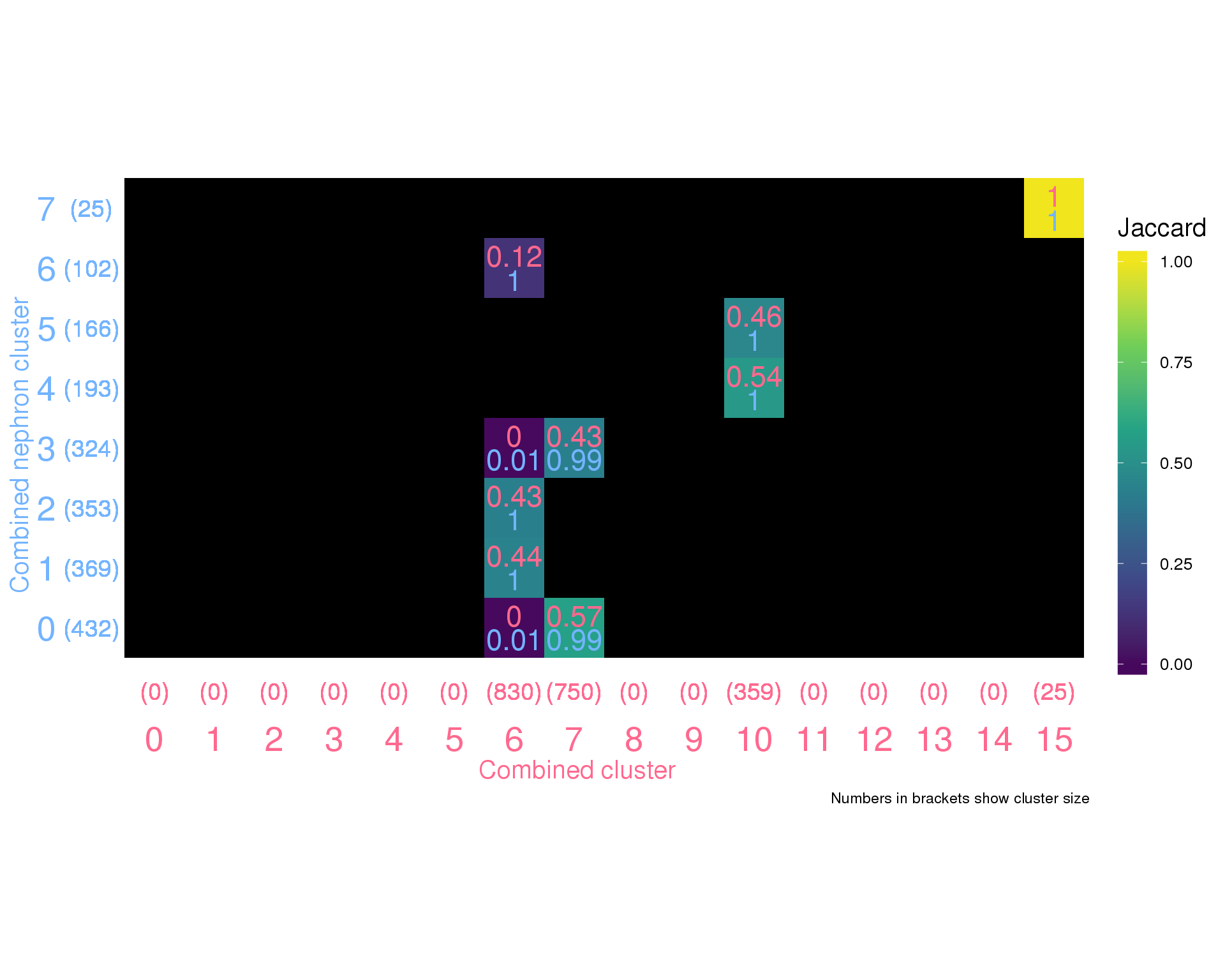Expand here to see past versions of combined-comb-neph-1.png:
Version Author Date
91ebd58 Luke Zappia 2018-11-21

## vs Organoids

summariseClusts(clusts, Combined, Organoids) %>%
ggplot(aes(x = Combined, y = Organoids, fill = Jaccard)) +
geom_tile() +
geom_text(aes(label = round(CombinedPct, 2)), nudge_y = 0.2,
colour = "#ff698f", size = 4) +
geom_text(aes(label = round(OrganoidsPct, 2)), nudge_y = -0.2,
colour = "#73b4ff", size = 4) +
geom_text(aes(label = glue("({CombinedTotal})")), y = -0.05,
size = 4, colour = "#ff698f") +
geom_text(aes(label = glue("({OrganoidsTotal})")), x = -0.05,
size = 4, colour = "#73b4ff") +
scale_fill_viridis_c(begin = 0.02, end = 0.98, na.value = "black",
limits = c(0, 1)) +
coord_equal() +
expand_limits(x = -0.5, y = -0.5) +
labs(x = "Combined cluster",
y = "Organoids cluster",
caption = "Numbers in brackets show cluster size") +
theme_minimal() +
theme(axis.text = element_text(size = 20),
axis.text.x = element_text(colour = "#ff698f"),
axis.text.y = element_text(colour = "#73b4ff"),
axis.ticks = element_blank(),
axis.title = element_text(size = 15),
axis.title.x = element_text(colour = "#ff698f"),
axis.title.y = element_text(colour = "#73b4ff"),
legend.key.height = unit(50, "pt"),
legend.title = element_text(size = 15),
legend.text = element_text(size = 10),
panel.grid = element_blank())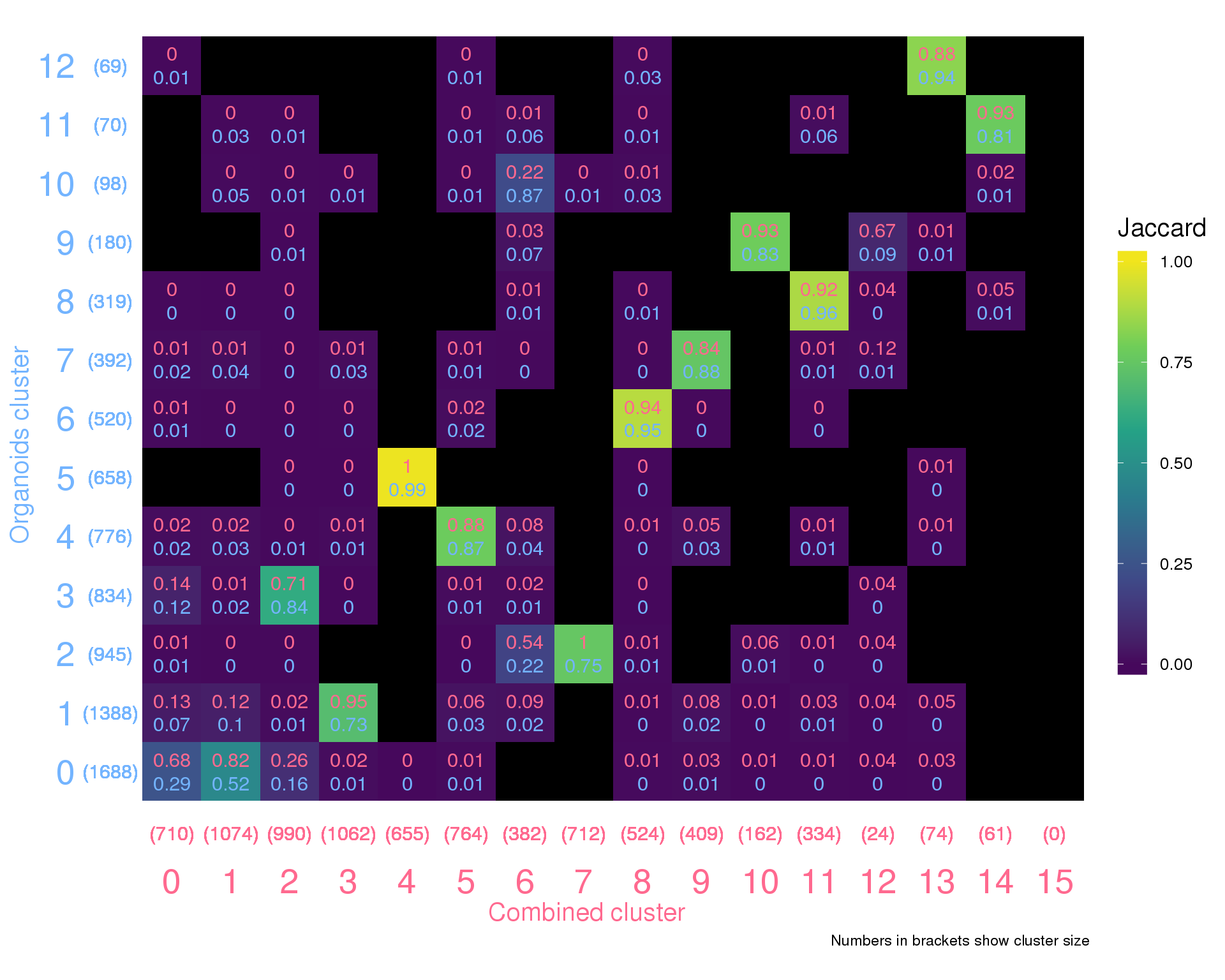Expand here to see past versions of combined-orgs-1.png:
Version Author Date
91ebd58 Luke Zappia 2018-11-21

## vs Organoids Nephron

summariseClusts(clusts, Combined, OrgsNephron) %>%
ggplot(aes(x = Combined, y = OrgsNephron, fill = Jaccard)) +
geom_tile() +
geom_text(aes(label = round(CombinedPct, 2)), nudge_y = 0.2,
colour = "#ff698f", size = 6) +
geom_text(aes(label = round(OrgsNephronPct, 2)), nudge_y = -0.2,
colour = "#73b4ff", size = 6) +
geom_text(aes(label = glue("({CombinedTotal})")), y = -0.05,
size = 5, colour = "#ff698f") +
geom_text(aes(label = glue("({OrgsNephronTotal})")), x = -0.05,
size = 5, colour = "#73b4ff") +
scale_fill_viridis_c(begin = 0.02, end = 0.98, na.value = "black",
limits = c(0, 1)) +
coord_equal() +
expand_limits(x = -0.5, y = -0.5) +
labs(x = "Combined cluster",
y = "Organoids nephron cluster",
caption = "Numbers in brackets show cluster size") +
theme_minimal() +
theme(axis.text = element_text(size = 20),
axis.text.x = element_text(colour = "#ff698f"),
axis.text.y = element_text(colour = "#73b4ff"),
axis.ticks = element_blank(),
axis.title = element_text(size = 15),
axis.title.x = element_text(colour = "#ff698f"),
axis.title.y = element_text(colour = "#73b4ff"),
legend.key.height = unit(50, "pt"),
legend.title = element_text(size = 15),
legend.text = element_text(size = 10),
panel.grid = element_blank())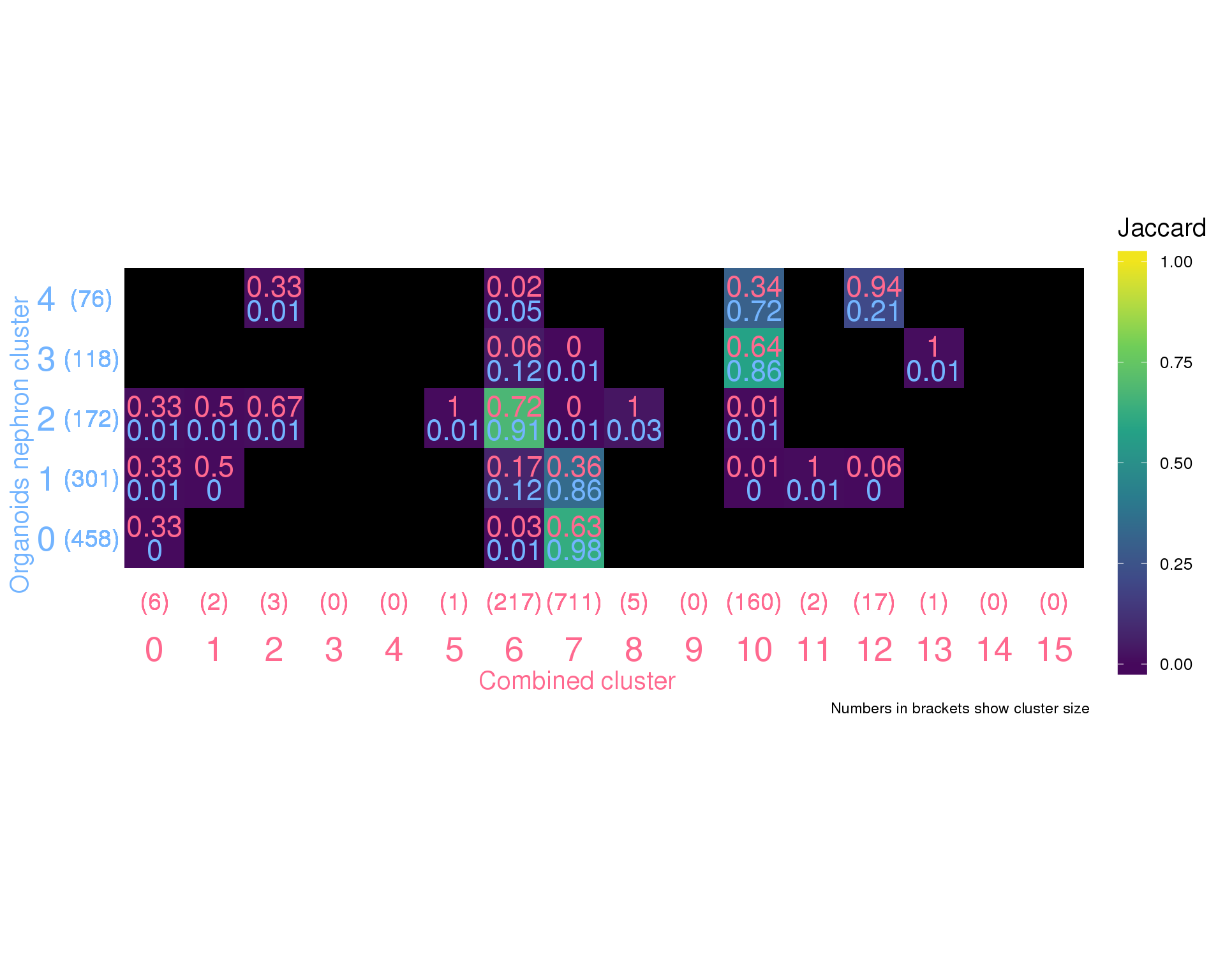Expand here to see past versions of combined-orgs-neph-1.png:
Version Author Date
91ebd58 Luke Zappia 2018-11-21

# Combined nephron

## vs Organoids Nephron

summariseClusts(clusts, CombNephron, OrgsNephron) %>%
ggplot(aes(x = CombNephron, y = OrgsNephron, fill = Jaccard)) +
geom_tile() +
geom_text(aes(label = round(CombNephronPct, 2)), nudge_y = 0.2,
colour = "#ff698f", size = 6) +
geom_text(aes(label = round(OrgsNephronPct, 2)), nudge_y = -0.2,
colour = "#73b4ff", size = 6) +
geom_text(aes(label = glue("({CombNephronTotal})")), y = -0.05,
size = 5, colour = "#ff698f") +
geom_text(aes(label = glue("({OrgsNephronTotal})")), x = -0.05,
size = 5, colour = "#73b4ff") +
scale_fill_viridis_c(begin = 0.02, end = 0.98, na.value = "black",
limits = c(0, 1)) +
coord_equal() +
expand_limits(x = -0.5, y = -0.5) +
labs(x = "Combined nephron cluster",
y = "Organoids nephron cluster",
caption = "Numbers in brackets show cluster size") +
theme_minimal() +
theme(axis.text = element_text(size = 20),
axis.text.x = element_text(colour = "#ff698f"),
axis.text.y = element_text(colour = "#73b4ff"),
axis.ticks = element_blank(),
axis.title = element_text(size = 15),
axis.title.x = element_text(colour = "#ff698f"),
axis.title.y = element_text(colour = "#73b4ff"),
legend.key.height = unit(50, "pt"),
legend.title = element_text(size = 15),
legend.text = element_text(size = 10),
panel.grid = element_blank())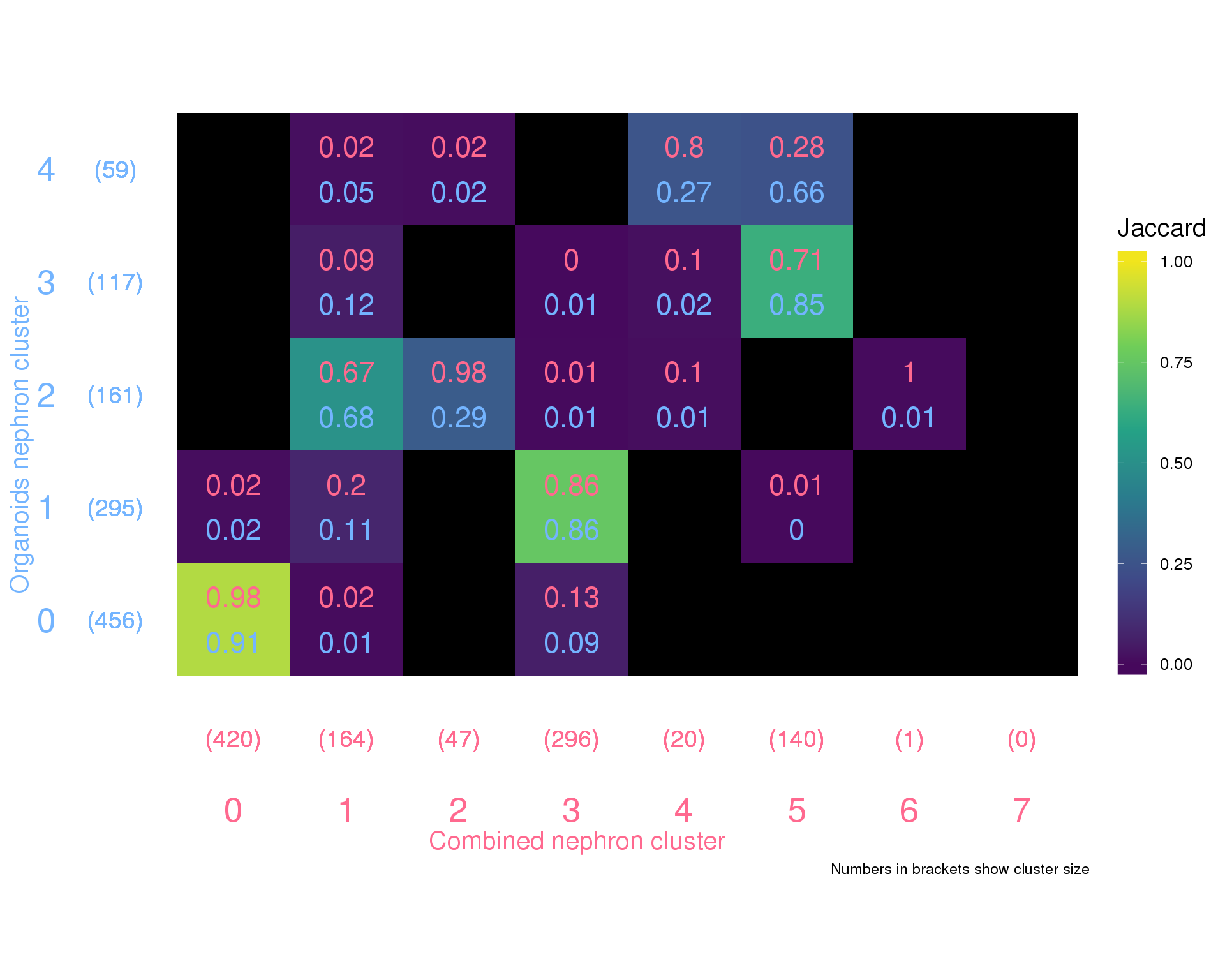Expand here to see past versions of comb-neph-orgs-neph-1.png:
Version Author Date
91ebd58 Luke Zappia 2018-11-21

# Summary

## Output files

This table describes the output files produced by this document. Right click and Save Link As… to download the results.

dir.create(here("output", DOCNAME), showWarnings = FALSE)

write_csv(clusts, here("output", DOCNAME, "cluster_assignments.csv"))

kable(data.frame(
File = c(
glue("[cluster_assignments.csv]",
),
Description = c(
"Cluster assignments for all clustering analyses"
)
))
File Description
cluster_assignments.csv Cluster assignments for all clustering analyses

## Session information

devtools::session_info()
 setting  value
version  R version 3.5.0 (2018-04-23)
system   x86_64, linux-gnu
ui       X11
language (EN)
collate  en_US.UTF-8
tz       Australia/Melbourne
date     2018-11-23

package     * version date       source
assertthat    0.2.0   2017-04-11 CRAN (R 3.5.0)
backports     1.1.2   2017-12-13 CRAN (R 3.5.0)
base        * 3.5.0   2018-06-18 local
bindr         0.1.1   2018-03-13 cran (@0.1.1)
bindrcpp    * 0.2.2   2018-03-29 cran (@0.2.2)
broom         0.5.0   2018-07-17 cran (@0.5.0)
cellranger    1.1.0   2016-07-27 CRAN (R 3.5.0)
cli           1.0.0   2017-11-05 CRAN (R 3.5.0)
colorspace    1.3-2   2016-12-14 cran (@1.3-2)
compiler      3.5.0   2018-06-18 local
crayon        1.3.4   2017-09-16 CRAN (R 3.5.0)
datasets    * 3.5.0   2018-06-18 local
devtools      1.13.6  2018-06-27 CRAN (R 3.5.0)
digest        0.6.15  2018-01-28 CRAN (R 3.5.0)
dplyr       * 0.7.6   2018-06-29 cran (@0.7.6)
evaluate      0.10.1  2017-06-24 CRAN (R 3.5.0)
forcats     * 0.3.0   2018-02-19 CRAN (R 3.5.0)
ggplot2     * 3.0.0   2018-07-03 cran (@3.0.0)
git2r         0.21.0  2018-01-04 CRAN (R 3.5.0)
glue        * 1.3.0   2018-07-17 cran (@1.3.0)
graphics    * 3.5.0   2018-06-18 local
grDevices   * 3.5.0   2018-06-18 local
grid          3.5.0   2018-06-18 local
gtable        0.2.0   2016-02-26 cran (@0.2.0)
haven         1.1.2   2018-06-27 CRAN (R 3.5.0)
here        * 0.1     2017-05-28 CRAN (R 3.5.0)
highr         0.7     2018-06-09 CRAN (R 3.5.0)
hms           0.4.2   2018-03-10 CRAN (R 3.5.0)
htmltools     0.3.6   2017-04-28 CRAN (R 3.5.0)
httr          1.3.1   2017-08-20 CRAN (R 3.5.0)
jsonlite      1.5     2017-06-01 CRAN (R 3.5.0)
knitr       * 1.20    2018-02-20 CRAN (R 3.5.0)
labeling      0.3     2014-08-23 cran (@0.3)
lattice       0.20-35 2017-03-25 CRAN (R 3.5.0)
lazyeval      0.2.1   2017-10-29 cran (@0.2.1)
lubridate     1.7.4   2018-04-11 cran (@1.7.4)
magrittr      1.5     2014-11-22 CRAN (R 3.5.0)
memoise       1.1.0   2017-04-21 CRAN (R 3.5.0)
methods     * 3.5.0   2018-06-18 local
modelr        0.1.2   2018-05-11 CRAN (R 3.5.0)
munsell       0.5.0   2018-06-12 cran (@0.5.0)
nlme          3.1-137 2018-04-07 CRAN (R 3.5.0)
pillar        1.3.0   2018-07-14 cran (@1.3.0)
pkgconfig     2.0.1   2017-03-21 cran (@2.0.1)
plyr          1.8.4   2016-06-08 cran (@1.8.4)
purrr       * 0.2.5   2018-05-29 cran (@0.2.5)
R.methodsS3   1.7.1   2016-02-16 CRAN (R 3.5.0)
R.oo          1.22.0  2018-04-22 CRAN (R 3.5.0)
R.utils       2.6.0   2017-11-05 CRAN (R 3.5.0)
R6            2.2.2   2017-06-17 CRAN (R 3.5.0)
Rcpp          0.12.18 2018-07-23 cran (@0.12.18)
readr       * 1.1.1   2017-05-16 CRAN (R 3.5.0)
readxl        1.1.0   2018-04-20 CRAN (R 3.5.0)
rlang         0.2.1   2018-05-30 CRAN (R 3.5.0)
rmarkdown     1.10.2  2018-07-30 Github (rstudio/rmarkdown@18207b9)
rprojroot     1.3-2   2018-01-03 CRAN (R 3.5.0)
rstudioapi    0.7     2017-09-07 CRAN (R 3.5.0)
rvest         0.3.2   2016-06-17 CRAN (R 3.5.0)
scales        0.5.0   2017-08-24 cran (@0.5.0)
stats       * 3.5.0   2018-06-18 local
stringi       1.2.4   2018-07-20 cran (@1.2.4)
stringr     * 1.3.1   2018-05-10 CRAN (R 3.5.0)
tibble      * 1.4.2   2018-01-22 cran (@1.4.2)
tidyr       * 0.8.1   2018-05-18 cran (@0.8.1)
tidyselect    0.2.4   2018-02-26 cran (@0.2.4)
tidyverse   * 1.2.1   2017-11-14 CRAN (R 3.5.0)
tools         3.5.0   2018-06-18 local
utils       * 3.5.0   2018-06-18 local
viridisLite   0.3.0   2018-02-01 cran (@0.3.0)
whisker       0.3-2   2013-04-28 CRAN (R 3.5.0)
withr         2.1.2   2018-03-15 CRAN (R 3.5.0)
workflowr     1.1.1   2018-07-06 CRAN (R 3.5.0)
xml2          1.2.0   2018-01-24 CRAN (R 3.5.0)
yaml          2.2.0   2018-07-25 cran (@2.2.0)                     

This reproducible R Markdown analysis was created with workflowr 1.1.1